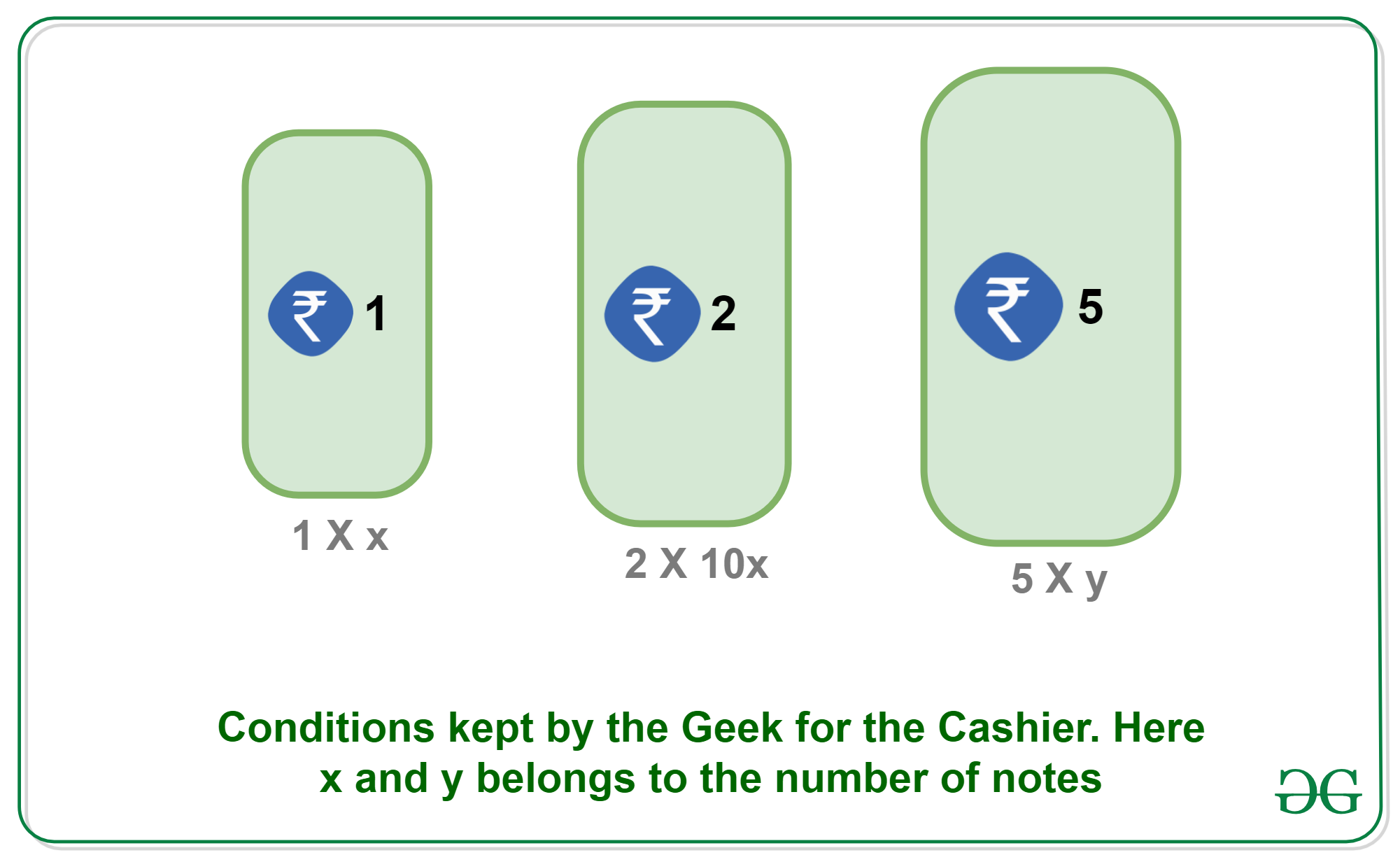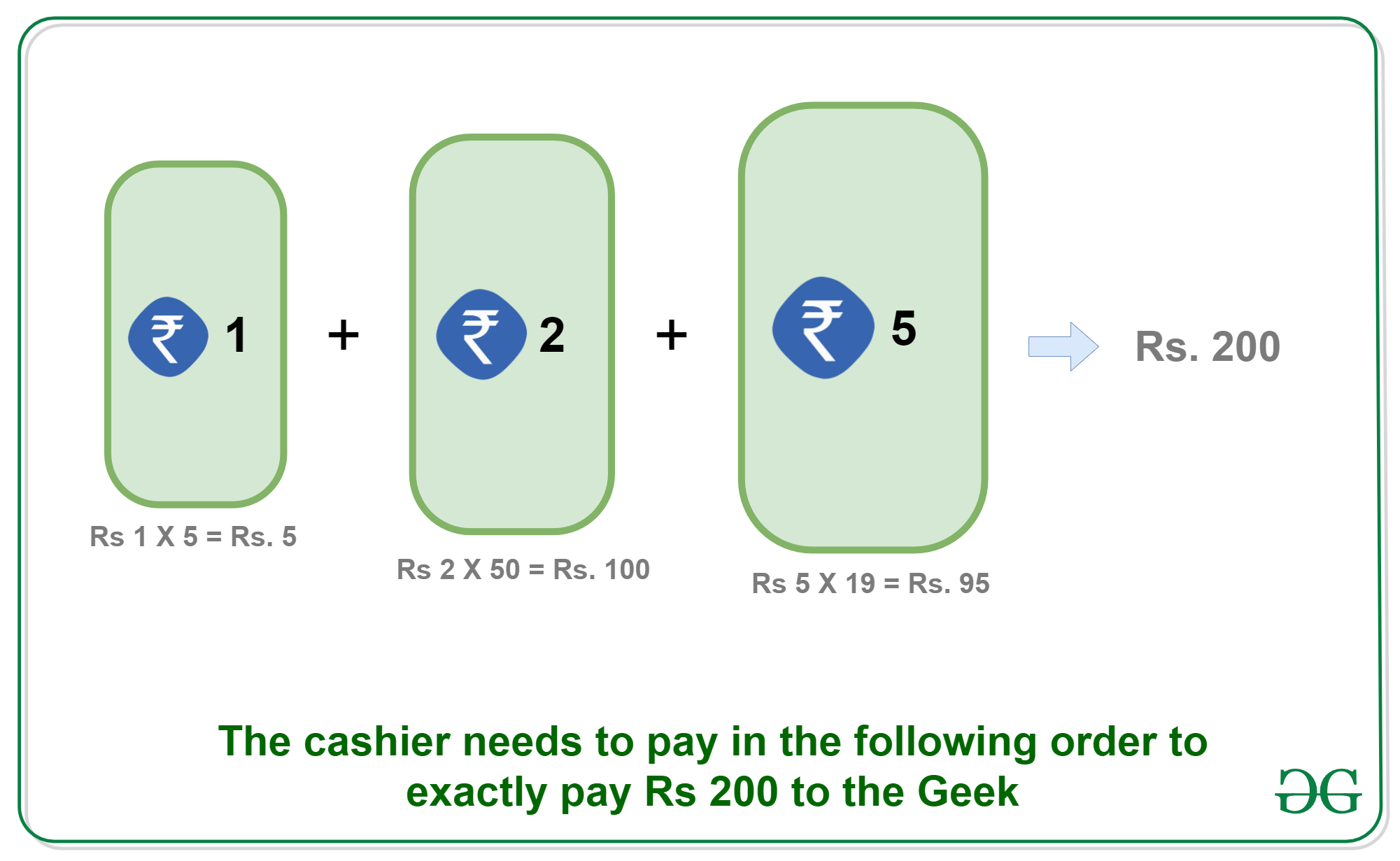Skip to content
Related Articles
Puzzle 55 | Geek and Cashier
• Difficulty Level : Basic
• Last Updated : 12 Dec, 2019

Puzzle: A Geek asks a cashier to pay Rs 200 for a cool program written by him. He asks the cashier to pay only in the following way:

1. Few 1 Rs Notes. Let x.
2. Few 2 Rs Notes. Must ten times of 1 Rs Notes, i.e., 10x.
3. Rest of the money as 5 Rs Notes(Minimum number Rs 5 notes should be used)How does the Geek’s Cashier plan to pay?

Solution: Let’s solve this in a stepwise manner:
The smallest amount of 1 Rs and 2 Rs notes the cashier may pay is 21 (1 one rupee note + 10 two rupees notes). So the cashier must pay in multiples of 21 to satisfy the first two conditions and also the amount must not exceed the total Rs. 200.

Multiples of 21 are:

`21, 42, 63, 84, 105, 126, 147 and 189.`

Out of this, only 105 is the multiple of 5. So he must give the balance of 95 in Rs 5 bills.Therefore, the Cashier must give 5 one-Rs bills, 50 two-Rs bills and 19 five-Rs bills.

This Puzzle is contributed by Cassin Thangam. Please write comments if you find anything incorrect, or you want to share more information about the topic discussed above.

My Personal Notes arrow_drop_up